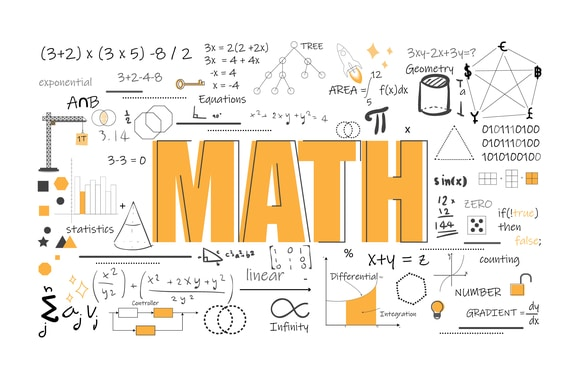# Numbers In Base Two II

Welcome to Class !!

In today’s Mathematics class, We will be looking at Multiplication and Division in Base Two. We hope you enjoy the class!#### MULTIPLICATION AND DIVISION IN BASE TWO

In multiplication, 0 x 0 = 0, 1 x 0 = 0, 1 x 1 = 1.

When there is long multiplication of binary numbers, the principle of addition can be used to derive the answer. Under division, the principle of subtraction can be used.

Worked Examples:

1. 1110 x 111

Solution:

1. Multiply 11.01 by 1.1 in base two

Solution:

##### DIVISION IN BASE TWO

Example:

Divide 110010101 by 1111

Solution:

1100101012  ÷  11112 = 110112

Example:

Find the square root of 10100012

Solution:

First convert it to base 10

10100012 = 1 x 2 6+ 0 + 1 x 2 4+ 0 + 0 + 0 + 1 x 20

= 64 + 16 + 1 = 81ten

The square root of 81 = 9ten

2 |  9       1

2 |  4       0

2 |  2       0

2 |  1     1

0 |  0

= 10012

EVALUATION

1. 10111÷110
2. 10001 x 11

We have come to the end of this class. We do hope you enjoyed the class?

Should you have any further question, feel free to ask in the comment section below and trust us to respond as soon as possible.

In our next class, we will be talking about Rational and Non-Rational Numbers Variation. We are very much eager to meet you there.

Are you a Parent? Share your quick opinion and win free 2-month Premium Subscription

Don`t copy text!